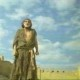• ## Java Binary to Decimal Conversion

Home » Forums » Developers, developers, developers » DevOps Lounge » Java Binary to Decimal Conversion

Tags:

Author
Topic
#2504053

I’m almost finished writing code that allows a user to enter a decimal number into binary, have the binary value reversed, and then convert it back to decimal.

Except for the Binary to Decimal phase, I solved everything. No matter what I type, it continues returning the same number to me. I’m not sure why I attempted to grasp this blog but it didn’t assist me. Simply put, why is the last section of my code incorrect? I’m having difficulties determining the length of the array/string and then multiplying it by 2n, etc.

```package reversedBinary;
import java.util.Scanner;

public class ReversedBinary {

public static void main(String[] args) {
int number;

Scanner in = new Scanner(System.in);

System.out.println("Enter a positive integer");
number=in.nextInt();

if (number <0)
System.out.println("Error: Not a positive integer");
else {

System.out.print("Convert to binary is:");
System.out.print(binaryform(number));
}

}

private static Object binaryform(int number) {
int remainder;

if (number <=1) {
System.out.print(number);

}

remainder= number %2;
binaryform(number >>1);
System.out.print(remainder);

{
return null;
} } }```
Author
Replies
• #2504117

I know nothing about Java but I do know that conversion is built-in to PowerShell. Do you really need to do in Java?

Foe example:If this is of any interest then have a look at Use PowerShell to Easily Convert Decimal to Binary and Back.

Hope this helps…

###### 1 user thanked author for this post.satrow
• #2504135

It isn’t working because your binaryform subroutine isn’t actually doing any of the math required to actually convert the decimal number into a binary value!

It needs to keep dividing the decimal number by 2 and record the result of the division as a “string value” of either 1 or 0 (0 = no remainder, 1 = remainder) until the results of the final division is either 0 or 1.

The recorded results of each division must be added to a “string value” that’s what gets displayed as the binary value when the routine is done.

With your problem explained, it’d be “much simpler” to use Java’s Integer.toBinaryString command.

i.e.  replace System.out.print(binaryform(number)); with System.out.print(Integer.toBinaryString(number));

And remove the whole binaryform subroutine.

###### 2 users thanked author for this post.satrow,Sky